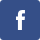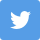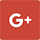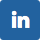Latest Results:

11 Plus LCM and HCF

11plusehelp.co.uk offers you unlimited online 11 Plus LCM and HCF maths tests.

LCM and HCF are among the most commonly used topic in mathematics. This is because they form the fundamentals for solving different quantitative problems. LCM stands Least common multiples. HCF stands Highest Common Factor.

Questions on this topic appear in the 11 plus entrance exams conducted by 11 plus grammar schools, independent schools and other private schools. This topic is external linking to solving times table, time and speed, time and work-related problems.

We need to look into the buzz words Factors and Multiples for a clear understanding of these concepts. HCF and LCM depend on prime factorization.

Factor:

The number that divides the given positive integer without leaving any remainder is called the factor.

For example, 24 ÷ 8 gives remainder=0.

Hence 8 is the factor of 24.

Multiple:

Multiple of a number is the result of multiplying a number by a whole number.

For example, the multiples of 4 can be obtained as

(4×1)=4, (4×2)=8,(4×3)=12,..... and so on.

Let us look at the definition of each acronym:

LCM:

It is an acronym for “ Least Common Multiple”. It is the least number which is exactly divisible by each of the given numbers.

HCF:

It is an acronym for “ Highest Common Factor “. It is the largest number that divides each of the given numbers. It is also called by the name GCD ( Greatest Common Divisor). It is calculated using factor trees.

The next question is how to compute them.

Finding Least Common Multiple LCM:

Given to find the LCM of 6 and 8, we proceed as follows:

List out the multiples of 6 and 8

The multiples of 6 are: 6×1=6, 6×2=12, 6×3=18, 6×4=24, 6×5=30,

The multiples of 8 are: 8×1=8, 8×2=16, 8×3=24, 8×4=32, 8×5=40,

The lowest and common multiple of both 6 and 8 is 24.

Hence LCM of 6 and 8 is 24.

Finding Highest Common Factor :

To compute the HCF of 6 and 12, we proceed as follows:

List out the factors of 6 and 12

The factors of 6 are: 1,2,3 and 6

The factors of 12 are: 1,2,3,4,6 and 12

The highest and common factor of both 6 and 12 is 6.

Hence LCM of 6 and 12 is 6.

Compute GCD and LCM using prime factors:

We employ this method when given numbers are large such that listing out all the factors and multiples for each number would be difficult.

Example problems of our website: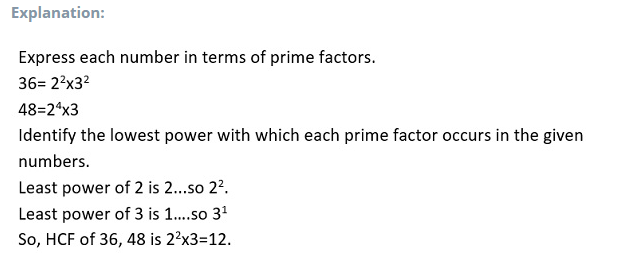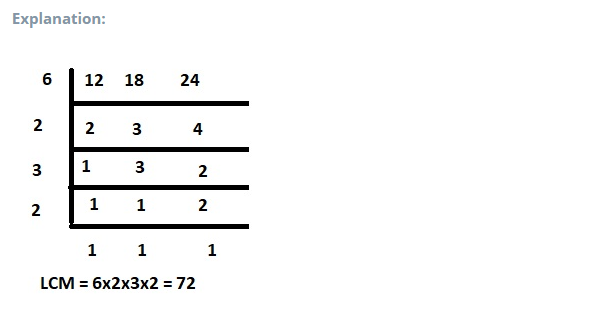Properties of LCM and HCF:

• The HCF of given numbers is not greater or more than any of the numbers.
• The LCM of given numbers is not less than any of the numbers.
• The HCF of two or more prime numbers is always 1.
• The LCM of two or more prime numbers is the product of them.
• The given two numbers, ‘p' and ‘q’, are equal to their HCF and LCM product. This means:

p × q = ( LCM of p and q ) × ( HCF of p and q )

• LCM of any fraction =   LCM of Numerators HCF of Denominators
• HCF of Fractions =  HCF of NumeratorsLCM of Denominators

Sample problems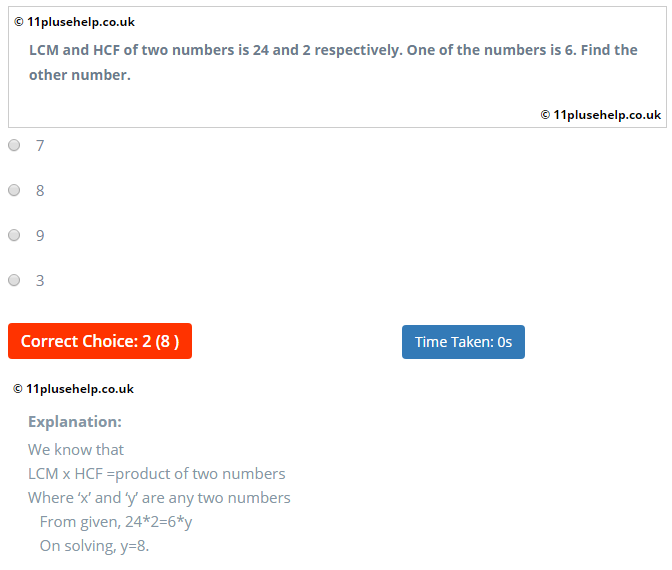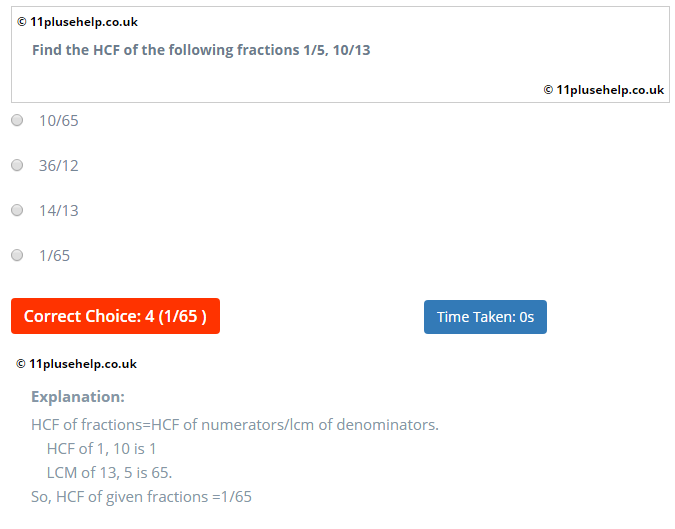You can access 11 Plus Maths Papers on different topics here:

https://www.11plusehelp.co.uk/11-plus-maths-practice-tests

11PluseHelp.co.uk is a one-stop-shop for 11 Plus exam preparation, including 11 Plus Independent schools preparation.

https://www.11plusehelp.co.uk/11-plus-topics/11-plus-maths-topics

We focus on fundamentals, logic, and basics and cover most of the UK syllabus for all 11 Plus exam patterns. For example, We cover Maths topic wise questions and fundamentals and how to apply them. We have continuously been adding 11 plus practice papers regularly.

You can access 11 Plus Practice Papers on different topics here:

https://www.11plusehelp.co.uk/11-plus-practice-papers

We have loads of questions useful for preparing for CEM, CSSE and GL assessment and any other 11 Plus entrance exams in the UK. Children will love to take these tests, and learning is fun at 11plusehelp.co.uk.

11 Plus Resources:

At 11plusehelp.co.uk/, we possess the following resources:

Maths- 11 Plus Maths Worksheets, 11 Plus Maths Papers free download, 11 Plus Maths topics pdfs.

English- 11 Plus English papers with answers, 11 Plus comprehension with answers, 11 English comprehensions multiple-choice, English reasoning test.

https://www.11plusehelp.co.uk/11-plus-topics/11-plus-english-topics

Verbal and Non-Verbal Reasoning- 11 Plus Verbal Reasoning worksheets free, Verbal and Non-Verbal Reasoning tests, easy Non-Verbal Reasoning Worksheets

You can access 11 Plus Verbal Reasoning Practice Papers on different topics here:

https://www.11plusehelp.co.uk/11-plus-practice-papers/11-plus-english-practice-test-papers/11-plus-verbal-practice-papers

You can access 11 Plus Non-Verbal Reasoning Practice Papers on different topics here:

https://www.11plusehelp.co.uk/11-plus-practice-papers/11-plus-english-practice-test-papers/11-plus-non-verbal-reasoning-practice-tests

Practice Tests- Eleven plus sample papers, free 11 plus papers with answers, 11 plus mock test papers pdf, 11 exam question and answers, UK 11 plus exam questions, 11 online tests in Maths and English with 11 Plus answers.

https://www.11plusehelp.co.uk/list-of-grammar-schools

https://www.11plusehelp.co.uk/top-100-independent-schools

11 Plus Revision Pack:

https://www.11plusehelp.co.uk/11-plus-practice-papers/11-plus-english-practice-test-papers/revision-pack

11PluseHelp.co.uk helps your children overcome the maths test's difficulty and top in the different school entrance exams. We provide online 11 plus maths tests designed in three mediums: Easy, Medium, and Difficult to prepare every child from basics to advanced level.

https://11plus-mockexams.co.uk/

You can access 11 Plus Exam FREE Papers by visiting the below link:
https://www.11plusehelp.co.uk/11-plus-free-online-papers

You can access 11 Plus Exam FREE Sample Papers by visiting the below link:
https://www.11plusehelp.co.uk/11-plus-sample-papers

11 Plus complete solution features:

https://11plusehelp.co.uk/blog/2017/05/01/11-plus-complete-solution/

Practice and Perseverance Over Genius and Talent

Thanks,

11Plusehelp.co.uk